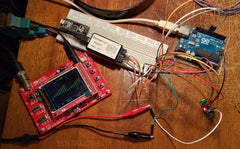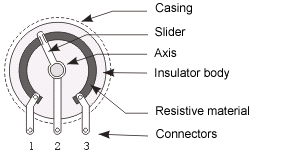# 🎛 How to connect the digital potentiometer X9C102, X9C103, X9C104 to Arduino

Consider the management of a digital potentiometer X9C (X9C102, X9C103, X9C503, X9C104) with the help of Arduino, as well as what areas of application this device may have. Let's use the ready module which costs less than 1 dollar.

Description of digital potentiometer type X9C

A potentiometer, or a variable resistor, is an electrical device that allows you to change the resistance of an electric current. The classical (mechanical) potentiometer consists of two terminals, between which the third is located - the movable ("sliding"). Moving the moving pin, we change the resistance between it and each of the fixed pin.An electronic potentiometer is an analog of a mechanical potentiometer, but with a number of advantages: it has no mechanical parts, it can be controlled remotely using, for example, a microcontroller, and it is significantly smaller in size.

Potentiometers are widely used in various electronic devices where it is necessary to adjust the voltage during operation. For example, as trimmers when setting up circuits, as volume controls in audio devices or lighting controls in lighting devices.

We will use a ready-made module with a digital potentiometer X9C102 (X9C103, X9C104, X9C503). Chinese friends sell them for less than 2 dollars.Digital potentiometer type X9C can be one of the following ratings:

 Name Maximum resistance X9C102 1 kOhm X9C103 10 kOhm X9C503 50 kOhm X9C104 100 kOhm

In the name of the potentiometer X9C, three digits mean the value and the number of zeros, which must be attributed to the value to get the nominal value. For example: 102 is 10 and 2 zeros, or 1000 ohms (1 kΩ); 503 is 50 and 3 zeros, or 50,000 (50 kΩ) etc.

The logic of the work and the connection diagram of the digital potentiometer X9C103 to the Arduino

Between 0 and the maximum value with a step of 1/100 of the maximum, you can adjust the resistance at the third "movable" output.

The control of the position of the "movable" output is carried out using a series of negative impulses. Each pulse shifts the resistance value by 1 step upwards or downwards.

The potentiometer is controlled by three lines:

Output name Purpose Note
CS Device selection LOW - the device is active
INC Output resistance change Negative impulses
U/D Direction of change U (up) - if the voltage on the chip leg HIGH, D (down) - LOW

Here is the timing diagram of control signals:Here, VW is the voltage at the center pin.
Let's assemble the circuit as shown in the figure:The module requires power +5 V.

Sketch control digital potentiometer X9C102, X9C103, X9C104

Now let's write this sketch:

```const int CS = 10;
const int INC = 9;
const int UD = 8;

void setup() {
pinMode(CS, OUTPUT);
pinMode(INC, OUTPUT);
pinMode(UD, OUTPUT);
digitalWrite(CS, HIGH);
digitalWrite(INC, HIGH);
digitalWrite(UD, HIGH);
}

void loop() {
for (int i=0; i<=100; i+=10) {
setResistance(i);
delay(100);
}
}

void setResistance(int percent) {
digitalWrite(UD, LOW);
digitalWrite(CS, LOW);
for (int i=0; i<100; i++) {
digitalWrite(INC, LOW);
delayMicroseconds(1);
digitalWrite(INC, HIGH);
delayMicroseconds(1);
}

digitalWrite(UD, HIGH);
for (int i=0; i<percent; i++) {
digitalWrite(INC, LOW);
delayMicroseconds(1);
digitalWrite(INC, HIGH);
delayMicroseconds(1);
}

digitalWrite(CS, HIGH);
}```

This sketch contains the following algorithm: we increase every 100 ms in 10% increments from 0 to 100% of the potentiometer maximum.

Load this sketch into the memory of the Arduino board.

Operation check digital potentiometer X9C102 / 103/104

With the help of a logic analyzer, let's see if it was possible to observe the timing diagram of the potentiometer control:It can be seen that completely. We lower the CS line to LOW, and also U / D to LOW (decrease in output resistance). When 100 pulses are counted on the INC, raise the U / D to HIGH (change the resistance upwards). With the help of INC with respect to the set zero resistance, we begin to count the desired value (in this case, 10 pulses equal to 10% of the maximum of the potentiometer).

Potentiometer X9C102 / 103/104 has 100 gradations of resistance between the minimum and maximum. This allows not to introduce any coefficients for converting interest into impulses. For example, 10 INC pulses change the current value of the output resistance by 10%.

If now with the help of a multimeter to check the resistance between the central and one of the final conclusions, then we will fix the change in resistance.

For clarity, I will apply a voltage of 5 volts between the end terminals of the potentiometer, and I will connect an oscilloscope to the center contact. The photos and videos below illustrate the result.